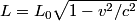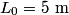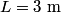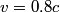## Solution to 1986 Problem 69

 The formula for the relativistic length contraction is:\begin{align*}L = L_0 \sqrt{1 - v^2/c^2}\end{align*}Plug in$L_0 = 5 \mbox{ m}$ and$L = 3 \mbox{ m}$ and solve for$v$ to find that$v = 0.8 c$. Hence answer (C) is correct.